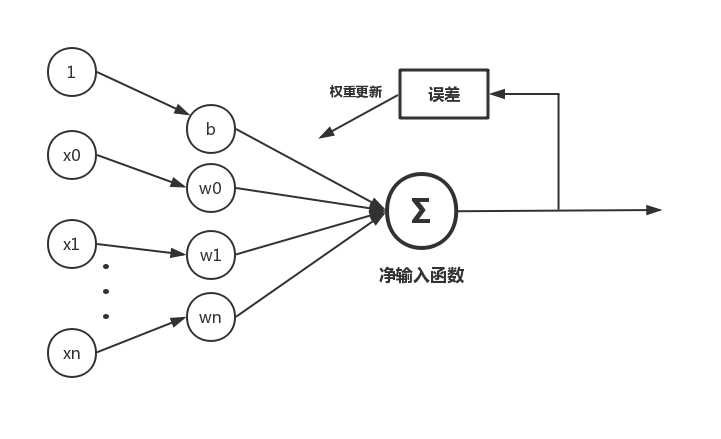max ide 示意圖 res tree near main atp then

# LinearRegression（線性回歸）

## 1.線性回歸簡介

#### 線性回歸定義：

百科中解釋

y：我們需要預測的數值；

w：模型的參數（即我們需要通過訓練調整的的值）

x：已知的特征值

b：模型的偏移量

#### 分類：

線性回歸屬於監督學習中的回歸算法；

線性回歸作為機器學習的入門級算法，很適合剛接觸機器學習的新手。雖然線性回歸本身比較簡單，但是麻雀雖小，五臟俱全，其中涉及到的“線性模型”、“目標函數”、“梯度下降”、“叠代”、“評價準則”等思想與其他復雜的機器學習算法是相通的，深入理解線性回歸後可以幫助你更加輕松的學習其他機器學習算法。

## 2.線性回歸模型解析

### 2.1 線性回歸模型示意圖### 2.2模型的組成部件

#### 2.2.1 假設函數（Hypothesis function）

$h_w(x) = b + w_0x_0 + w_1x_1 + ··· +w_nx_n$

使用向量方式表示：

$X=\begin{bmatrix} \ x_0 \\ x_1 \\\vdots \\ x_n \end{bmatrix},W=\begin{bmatrix} \ w_0 \\ w_1 \\\vdots \\ w_n \end{bmatrix}$

則有：$h_w(x) = W^TX+ b$

#### 2.2.2 損失函數：（Cost function）

這裏使用平方差作為模型的代價函數

$J(w) = \frac{1}{2m}\sum_{i=1}^{m}(h_w(x^{(i)}) - y^{(i)})^2$

#### 2.2.3 目標函數：（Goal function）

$minimize(J(w))$

#### 2.2.4 優化算法：(optimization algorithm)

關於梯度下降法這裏不詳細介紹；

## 3.使用python實現線性回歸算法1 #-*- coding: utf-8 -*-
2 import numpy as np
3 from matplotlib import pyplot as plt
4
5
6 #生成訓練使用數據;這裏線性函數為 y = 1.5*x + 1.3
7 def data_generate():
8     #隨機生成100個數據
9     x = np.random.randn(100)
10     theta = 0.5   #誤差系數
11     #為數據添加幹擾
12     y = 1.5*x + 1.3 + theta*np.random.randn(100)
13     return x,y
14
15 class LinearRegression():
16     ‘‘‘
17     線性回歸類
18     參數：
19         alpha:叠代步長
20         n_iter:叠代次數
21     使用示例：
22         lr = LinearRegression() #實例化類
23         lr.fit(X_train,y_train) #訓練模型
24         y_predict = lr.predict(X_test) #預測訓練數據
25         lr.plotFigure()用於畫出樣本散點圖與預測模型
26     ‘‘‘
27     def __init__(self,alpha=0.02,n_iter=1000):
28         self._alpha = alpha     #步長
29         self._n_iter = n_iter    #最大叠代次數
30
31     #初始化模型參數
32     def initialPara(self):
33         #初始化w，b均為0
34         return 0,0
35
36     #訓練模型
37     def fit(self,X_train,y_train):
38         #保存原始數據
39         self.X_source = X_train.copy()
40         self.y_source = y_train.copy()
41
42         #獲取訓練樣本個數
43         sample_num = X_train.shape
44         # 初始化w，w0
45         self._w, self._b = self.initialPara()
46
47         #創建列表存放每次每次叠代後的損失值
48         self.cost = []
49
50         #開始訓練叠代
51         for _ in range(self._n_iter):
52             y_predict = self.predict(X_train)
53             y_bias = y_train - y_predict
54             self.cost.append(np.dot(y_bias,y_bias)/(2 * sample_num))
55             self._w += self._alpha * np.dot(X_train.T,y_bias)/sample_num
56             self._b += self._alpha * np.sum(y_bias)/sample_num
57
58     def predict(self,X_test):
59         return self._w * X_test + self._b
60
61     #畫出樣本散點圖以及使用模型預測的線條
62     def plotFigure(self):
63         #樣本散點圖
64         plt.scatter(self.X_source,self.y_source,c=‘r‘,label="samples",linewidths=0.4)
65
66         #模型預測圖
67         x1_min = self.X_source.min()
68         x1_max = self.X_source.max()
69         X_predict = np.arange(x1_min,x1_max,step=0.01)
70         plt.legend(loc=‘upper left‘)
71
72         plt.plot(X_predict,self._w*X_predict+self._b)
73         plt.show()
74
75 if __name__ == ‘__main__‘:
76     #創建訓練數據
77     x_data,y_data = data_generate()
78
79     #使用線性回歸類生成模型
80     lr = LinearRegression()
81     lr.fit(x_data,y_data)
82
83     #打印出參數
84     print(lr._w,lr._b)
85     #畫出損失值隨叠代次數的變化圖
86     plt.plot(lr.cost)
87     plt.show()
88     #畫出樣本散點圖以及模型的預測圖
89     lr.plotFigure()
90
91     #預測x
92     x = np.array()
93     print("The input x is{0},then the predict of y is:{1}".format(x,lr.predict(x)))

Python機器學習/LinearRegression（線性回歸模型）（附源碼）